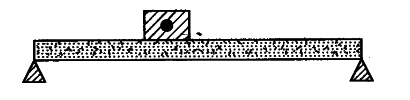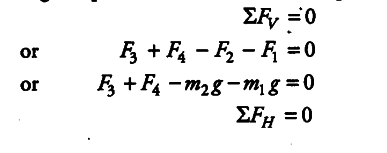# A beam of uniform cross-section and uniform mass-density of mass 20 kg is supported at ends

A beam of uniform cross-section and uniform mass-density of mass 20 kg is supported at ends. A mass of 5 kg is placed at a distance ofL/5 m from one of its end. If beam is L m long, what are reactions of supports?

In given case, our system look likeForces involved are (i) weight of beam (ii) weight of mass placed over beam (ii) Reactions of supports which we have to found.
Free body diagram of our system isIn static equilibrium problem, calculation involves following equations
Sum of all vertical (or vertical component of) forces is zero,
Sum of all horizontal forces or horizontal components of forces is zero,
Sum of all torques is zero.

You may take signs as
All forces along + y-direction are positive.
All forces along + x-direction are positive.
All forces along - y-direction are negative.
All forces along — x-direction are negative.
All anti-clockwise torques are positive.
All clockwise torques are negative.
In given problem, as the beam is in equilibrium